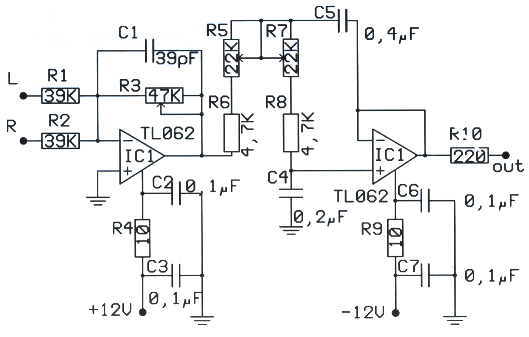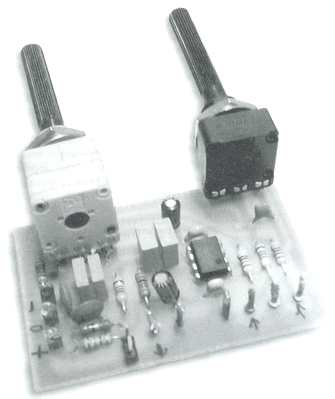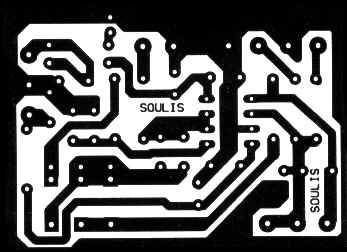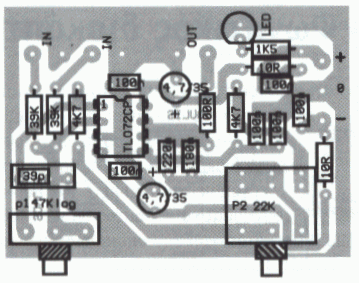# 低通滤波器 - 用于低音炮电路R1 = 39千欧
R2 = 39千欧
R3 = 47千欧
R4 = 10欧姆
R5 = 22千欧
R6 = 4,7千欧
R7 = 22千欧
R8 = 4,7千欧
R9 = 10欧姆
R10 = 220欧姆
C1 = 39 pF的
C2 = 0.1 uF的
C3 = 0.1 uF的
C4 = 0.2 uF的
C5 = 0.4 uF的
C6 = 0.1 uF的
C7 = 0.1 uF的
IC1 = TL064